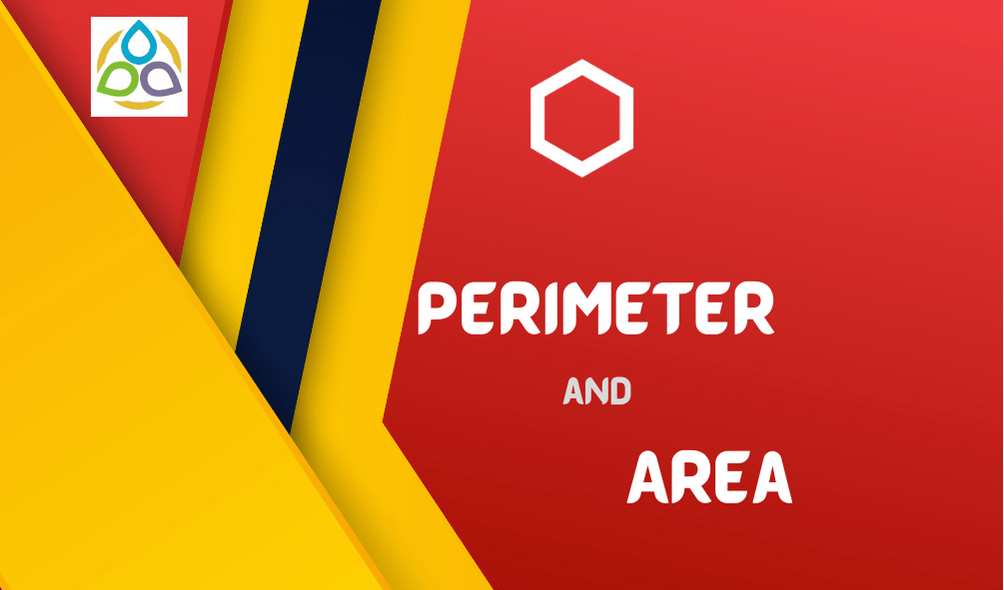# Perimeter and Area

16. Area of a rectangle and the area of a circle are equal. If the dimensions of the rectangle are 14cm × 11 cm, then radius of the circle is

• (a) 21 cm
• (b) 10.5 cm
• (c) 14 cm
• (d) 7 cm

15. In reference to a circle the value of π is equal to

• (a) area/ circumference
• (b) area/ diameter
• (c) circumference/ diameter

14. Circumference of a circle is always

• (a) more than three times of its diameter
• (b) three times of its diameter
• (c) less than three times of its diameter
• (d) three times of its radius

13. Circumference of a circle of diameter 5 cm is

• (a) 3.14 cm
• (b) 31.4 cm
• (c) 15.7 cm
• (d) 1.57 cm

12. Circumference of a circle disc is 88 cm. Its radius is

• (a) 8 cm
• (b) 11 cm
• (c) 14 cm
• (d) 44 cm

11. Area of circular garden with diameter 8 m is

• (a) 12.56 m2
• (b) 25.12 m2
• (c) 50.24 m2
• (d) 200.96 m2

10. Area of a circle with diameter m radius n and circumference p is

• (a) 2πn
• (b) πm2
• (c) πp2
• (d) πn2

9. A table top is semi-circular in shape with diameter 2.8 m. Area of this table top is

• (a) 3.08 m2
• (b) 6.16 m2
• (c) 12.32 m2
• (d) 24.64 m2

8. If radius of a circle is increased to twice its original length, how much will the area of the circle increase?

• (a) 1.4 times
• (b) 2 times
• (c) 3 times
• (d) 4 times

7. What will be the area of the largest square that can be cut out of a circle of radius 10 cm?

• (a) 100 cm2
• (b) 200 cm2
• (c) 300 cm2
• (d) 400 cm2

6. What is the radius of the largest circle that can be cut out of the rectangle measuring 10 cm in length and 8 cm in breadth?

• (a) 4 cm
• (b) 5 cm
• (c) 8 cm
• (d) 10 cm

5. The circumference of a circle whose area is 81πr2, is

• (a) 9πr
• (b) 18πr
• (c) 3πr
• (d) 81πr

4. The area of a square is 100 cm2. The circumference (in cm) of the largest circle cut of it is

• (a) 5π
• (b) 10π
• (c) 15π
• (d) 20π

3. If the radius of a circle is tripled, the area becomes

• (a) 9 times
• (b) 3 times
• (c) 6 times
• (d) 30 times

2. The area of a semicircle of radius 4r is

• (a) 8πr2
• (b) 4πr2
• (c) 12πr2
• (d) 2πr2

1. Length of tape required to cover the edges of a semi-circular disc of radius 10 cm is

• (a) 62.8 cm
• (b) 51.4 cm
• (c) 31.4 cm
• (d) 15.7 cm

1.Ty Hillesheim says:
2.zoritoler imol says: